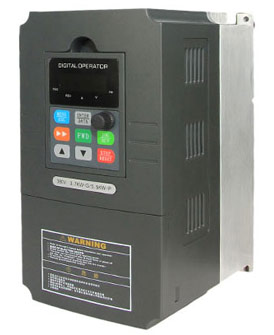Categories

# Does variable frequency drive have power factor?

I do not understand how Power Factor can be affected with typical DC drives, as power factor only exist in AC system when the current wave is either leading or lagging the voltage wave. For DC drives, since phase angles do not exist, why would power factor be affected? This is also my understanding on why power factor is not affected on the line side of variable frequency drive (VFD), as AC voltage is first converted into the DC link voltage before the IGBT converts the energy back to AC current. On the load side of the VFD, since the voltage is in a series of square waves, I don't know if "Power Factor" is still the appropriate term to explain the phase angle relationship between the voltage and current. Maybe power factor doesn't even exist at the load side of VFD, as voltage is now a series of DC square waves while current is a continuous sine wave.In the case of acceleration, I think it would affect power factor because the motor is operating at a higher slip compare to when it's operating at a constant load. As I understand, when the rotor of an induction motor is running at a speed lower than the synchronous speed (always), the power factor will be less than unity. During acceleration, higher slip is needed to provide additional torque to accelerate and this is my reason to think that power factor is affected during acceleration. In a typical servo control application, the induction motor is mostly operating in high slip mode, but most induction motor's rated power factor is based on the motor running at rated speed and rated torque under constant speed. Of course, I'm now thinking in terms of an induction motor directly powered by the line voltage, but these assumptions probably don't apply to an induction motor powered by a VFD for the reasons given above.

BTW, my VFDs are equipped with Active Line Modules, so they're able to perform regenerative power feedback.

Buy VFDs on GoHz.com, 1/2hp VFD, 2hp VFD, 5hp VFD...